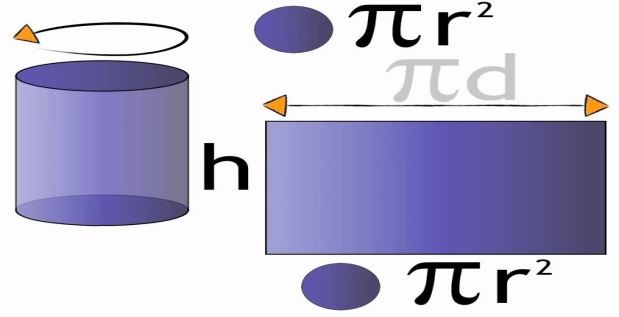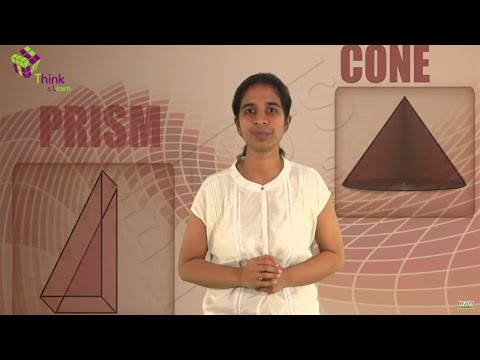Surface Area of a Cylinder

A cylinder is a three-dimensional structure having a circular base. A cylinder can be seen as a set of circular disks that are stacked on one another.  Since the cylinder is a three-dimensional shape,  it has a surface area and volume. The surface area of a cylinder can be classified into two types namely,

• Curved Surface Area (CSA)
• Total Surface Area (TSA)

Now, think of a scenario where we need to paint the faces of a cylindrical container. Before we start painting, we need to know the quantity of paint required for painting all the walls. Thus, we need to know the area of all the faces of this container in order to calculate the quantity of paint required. We define this term as the total surface area.

• The total surface area of a cylinder is equal to the sum of areas of all its faces.
• The curved surface area of a cylinder is defined as the area of the curved surface of a cylinder.

Let us now find out a general formula for the calculation of the surface area of a cylinder.

Surface Area of a Cylinder Formula

Let us take a cylinder of base radius ‘r’ and height ‘h’ units. The curved surface of this cylinder, if opened along the diameter (d=2r) of the circular base can be transformed into a rectangle of length ‘2πr’ and height ‘h’ units. Thus,Therefore, the Curved surface area of a cylinder of base radius ‘r’, and height ‘h’,

Curved Surface Area of a Cylinder= 2π × r × h Square units

The Total surface area of a cylinder of base radius ‘r’, and height ‘h’ = 2π × r × h + area of two circular bases,

Total Surface Area of a Cylinder= 2π × r × h + 2πr2= 2πr (h + r) Square unitsSurface Area of a Cylinder Problem

Question:

Calculate the cost required to paint a container which is in shape of a right circular cylinder having a base radius of 7 m and height 13 m. If the painting cost of the container is INR 2.5/m2. (Take π = 22/7)

Solution:

Total surface area of aquarium = 2πr (h + r)$= 2 \times \frac{22}{7} \times 7 \times 20$= 880 m2

Total cost of painting the container = 2.5 × 880 = Rs. 2200

To learn and practice more problems related to the calculation of surface area and volume of a cylinder, download BYJU’S – The Learning App.

 Related Links Volume of a Cylinder Volume of a Cone Volume of a Cube Volume of a sphere